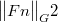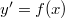ď»ż

### Introduzione

#### Abstract

En
In this note we observe a decreasing property of${\big \Vert {Fn} \big \Vert} _{G}2$ along the numerical solution of the autonomous differential system$y'=f(x)$ which satisfies a monotonicity condition;such a solution is obtained by means of a class of linear k-step A-stable methods and we have set(Error rendering LaTeX formula) and G is a symmetric positive definite matrix of order k. We study also a particular subclass of linear multistep G-stable methods of maximum order,in which the matrix G is actually constructed.The associated Lyapunov function ensures the stability of the set of equilibrium points.

DOI Code: §

Full Text: PDF